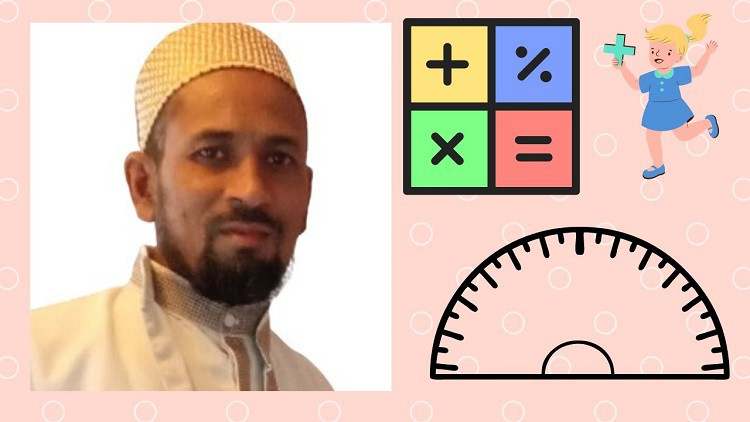# Operations of integersaddition and subtraction of integers, Multiplication and division of integers, BODMAS

What you will learn

### The most important concept BODMAS will help them to solve the questions easily and in interesting manner.

Description

This is the basic course of mathematics for the students to learn the basic concept. The students will learn the operations of integers. They will also learn the basic concept of BODMAS. This course will boost the confidence of the students to solve mathematical questions and they will learn to solve the problem easily. The students from standards 6 to standard 10 will learn the basic concept the would be able to get good marks in Mathematics. They will learn different types of numbers. operations of integers. They will learn to add and subtract the integers. The course will explain to multiply and divide integers. After completing the course they will solve the problem easily and in an interesting manner. This is an essential course for all students and they should learn this basic concept.

The course is divided into three sections. After every section, you will find a pdf to solve questions. download the pdf and solve the questions. After that, download the second pdf which will provide the answer key. Check your answer and if there is any mistake correct it.

If you have any doubt, you can ask me. I will try to solve your doubt and if necessary I will take  two or three doubt session online.

All the best

English
language

Content

### Different types of numbers

Introduction

Solution with example
Solution of questions

### Multiply and Division

Multiplication and division of integers

BODMAS
Solution

### Revision

What did you learn?